Your research can change the world
More on impact ›

# Frontiers in Applied Mathematicsand Statistics## ORIGINAL RESEARCH article

Front. Appl. Math. Stat., 23 June 2020 | https://doi.org/10.3389/fams.2020.00021

# A Finite Element Method for a Fourth Order Surface Equation With Application to the Onset of Cell Blebbing

• Mathematics Institute, University of Warwick, Coventry, United Kingdom

A variational problem for a fourth order parabolic surface partial differential equation is discussed. It contains non-linear lower order terms, on which we only make abstract assumptions, and which need to be defined for specified problems. We derive a semi-discrete scheme based on the surface finite element method, show a-priori error estimates, and use the analytical results to prove well-posedness. Furthermore, we present a computational framework where specific problems can be conveniently implemented and, later on, altered with relative ease. It uses a domain specific language implemented in Python. The high level program control can also be done within the Python scripting environment. The computationally expensive step of evolving the solution over time is carried out by binding to an efficient C++ software back-end. The study is motivated by cell blebbing, which can be instrumental for cell migration. Starting with a force balance for the cell membrane, we derive a continuum model for some mechanical and geometrical aspects of the onset of blebbing in a form that fits into the abstract framework. It is flexible in that it allows for amending force contributions related to membrane tension or the presence of linker molecules between membrane and cell cortex. Cell membrane geometries given in terms of a parameterization or obtained from image data can be accounted for by the software. The use of a domain specific language to describe the model makes is straightforward to add additional effects such as reaction-diffusion equations modeling some biochemistry on the cell membrane. Some numerical simulations illustrate the approach.

## 1. Introduction

We present and analyse a finite element approximation to parabolic fourth order surface partial differential equations of the form

for a vector field $u=\left({u}_{1},{u}_{2},{u}_{3}\right):{\Gamma }^{0}\to {ℝ}^{3}$ on a surface Γ0 that is the smooth boundary of a bounded domain in ℝ3. Here, ${\Delta }_{{\Gamma }^{0}}$ is the Laplace–Beltrami operator and ψ′ and k are given Lipschitz continuous functions (see section 2.2 for precise definitions and assumptions). We also present a software framework that facilitates a convenient implementation of specific problems and report on numerical simulations on an application in cell biology.

Our investigation is motivated by cell blebbing, which refers to the detachment of the plasma membrane from its actin cytoskeleton and the fast formation of a spherical protrusion. This is then followed by a slower reformation of the actin cortex close to the deformed part of the membrane and a retraction phase [1, 2]. The phenomenon is observed in various processes including apoptosis, spreading, migration, division, embryonic development, and viral entry (see Ponuwei  for a recent overview), and is the subject of significant ongoing research. In Tyson et al.  and Collier et al.  a mechanical model based on ideas in Young and Mitran  and Strychalski and Guy  for curves in 2D is presented. It accounts for membrane tension, excess cell pressure, membrane bending resistance, forces due to molecules connecting the membrane with the cortex, and drag due to motion through the viscous ambient fluid, and it is used to study the influence of the initial geometry on the propensity to nucleate blebs. One of our objectives is to extend this model to surfaces in 3D and to perform numerical simulations of the onset of blebbing.

Computational approaches to cell blebbing are based on various methodology for fluid-structure interaction. In 2D, the membrane may be tracked by a closed curve. Forces due to its elastic properties can be computed by approximating the curve with a polygonal chain and using finite difference techniques as in Tyson et al.  and Collier et al. . The approach can be interpreted as a finite element method and then analyzed in a variational framework. We aim for a generalization of this approach including its convergence analysis to surfaces in 3D that are approximated by triangulated surfaces. If (viscous) fluid flow inside and outside of the cell is accounted for then these membrane forces can be incorporated into the flow equations with a Dirac delta distribution. Smoothing the delta distribution according to certain principles and approximating the fluid flow equations on a regular bulk grid underpins the immersed boundary method, which is understood as both a mathematical formulation and a numerical approach . Based on these ideas, in Young and Mitran , a vorticity-stream formulation for the Newtonian, viscous flow is used, and in Strychalski and Guy , a staggered grid finite difference method. As an alternative, there are boundary element formulations that are set up directly on the polygonal chain . In 3D, the fluid flow becomes significantly more expensive, and tracking points on a surface whilst maintaining a good representation becomes much more involved. In Maxian et al. , a spectral method for the flow is coupled with two approaches for the surface, one based on a parameterization with spherical harmonics and one based on a piecewise linear representation. In Campbell and Bagchi [11, 12] a surface finite element method for reaction-diffusion equations on the cell membrane is coupled with a projection method for the flow. In both approaches the immersed boundary method is used for the coupling. Alternatively to tracking the membrane, interface capturing methods may be used. We are not aware of such an approach to cell blebbing but in Moure and Gomez  3D simulations of a phase field model for moving cells are presented, which uses isogeometric analysis for the spatial approximation and a second order stable time discretisation involving a two-stage predictor-corrector scheme.

The coupling between the surface terms and the bulk flow may also be realized using variational approaches that are amenable to finite element techniques. In the last chapter of Barrett et al.  this is presented for vesicles formed by biomembranes that are governed by the Helfrich energy . Such methods address the quality of the evolving surface mesh but a convergence analysis seems out of reach. However, accounting for the fluid flow with a simple drag force or just relaxing some elastic surface energy with a gradient flow dynamics leads to geometric evolution equations, and for the simplest one, the mean curvature flow, convergence of a surface finite element method has been proved recently . Cell blebbing will lead to a more complicated problem but as our focus is on the onset of blebbing, which involves only small deformations, we can expect reasonable results by parameterizing the membrane position over a reference surface. We choose the initial surface as a reference surface and show that the emerging PDE problem can then be of the form (1). This motivates one of our main objectives, namely to prove weak well-posedness and convergence of a surface finite element method for such type of problems. To this end, we proceed essentially as in Elliott and Ranner , where the Cahn–Hilliard equation, a scalar parabolic fourth order equation, on an evolving surface is analyzed. The procedure involves splitting the fourth-order problem into two equation of second order by introducing a support field, namely

$w=−ΔΓ0u,$

that can be interpreted as the curvature of the membrane. The essential challenge is the non-linear second order term in (1). To deal with it, we exploit the above linear equation to show strong convergence of the gradient of u.

The surface finite element method dates at least back to Dziuk  for the Laplace–Beltrami operator on stationary surfaces and has seen significant development since . It is a versatile tool that can be used for cell motility modeled by a geometric evolution equation coupled with a systems of surface reaction-diffusion equations  and can be coupled with bulk finite elements in one of the adjacent domains [21, 22] in a fitted approach in the sense that the surface mesh is the boundary of a bulk mesh. Also approaches for the unfitted case of a surface mesh intersecting a fixed bulk mesh rather arbitrarily have been developed, where we mention the trace finite element method  and the cut finite element method . In both methods, PDEs are solved on the intersection of a surface with a, usually, Cartesian bulk mesh on which additional terms are set up for stabilization to deal with small intersection patches. For the case of a PDE on an evolving surface the trace finite element method has been analyzed in depth . All these methods have in common that they are usually not provided by PDE software packages out of the box but require expert knowledge to implement them. One of our objectives is therefore to make the methodology we have developed accessible by providing code that enables users to implement specific problems with relative ease.

Let us briefly summarize our objectives and achievements, and outline the paper:

• The surface PDE (1) is analyzed for well-posedness using surface finite element techniques. Stability estimates for the semi-discrete scheme are derived and exploited to show convergence. Under slightly more restrictive assumptions on the quality of the solution, error estimates are shown. The suitable abstract variational problem is thoroughly formulated in section 2.2, and the finite element approach is presented and analyzed in section 3.

• The force balance model in Tyson et al.  and Collier et al. , which is based on ideas in Young and Mitran  and Strychalski and Guy , is extended from curves in 2D to surfaces in 3D. More precisely, a continuum model is presented such that, when restricting the model to a curve in 2D and discretising the governing equations using standard finite difference methods, the original computational model is obtained. This specific model has been used for some numerical simulations and is derived in section 4.

• A software framework for numerical simulations has been developed. It features a high-level interface to implement a problem in the Unified Form Language (UFL) , which enables a user to conveniently alter the variational problem. Whilst the overall program control and the time stepping are done at the high level, bindings to the Distributed Unified Numerics Environment (DUNE) [27, 28] are used for efficiently discretising and solving the spatial problems, more precisely, the Python bindings to the DUNE-FEM module [29, 30]. Section 5 contains the time discretisation, details on the implementation, and numerical simulations, which also demonstrate the extensibility of the software package.

## 2. Variational Problem Formulation

### 2.1. Setting and Notation

Let [0, T] for some T > 0 denote a time interval and let Ω(t), t ∈ [0, T], be an open, time dependent bounded domain. Its evolving boundary Γ(t) = ∂Ω(t) is parameterized over the initial (smooth) surface Γ0 = Γ(0), i.e., Γ(t) = u0, t) for some function u0 × [0, T] → ℝ3 such that $u\left(·,0\right)={id}_{{\Gamma }^{0}}$ is the identic map of Γ0. The setup is illustrated in Figure 1, which also shows some forces acting on the surface that are explained in section 4. The dependence on t will usually be dropped in the following. We furthermore introduce the following notation:

We aim for approximating the PDE problem (1) using finite elements and thus require a variational formulation. We say that a function fL10) has a weak derivative ${\eta }_{i}={\underset{_}{D}}_{i}f\in {L}^{1}\left({\Gamma }^{0}\right)$ if

holds true for all smooth functions φ with compact support. We also use ${\nabla }_{{\Gamma }^{0}}$ to denote this weak derivative and write ${\nabla }_{{\Gamma }^{0}}^{k}$, k ∈ ℕ, for the k-th derivative. Sobolev spaces on Γ0 are defined by H00) = L20) and

For a function η ∈ H1 and a vector field v${\left({L}^{2}\right)}^{3}$ the definition of the weak derivative yields that

For the L2 “mass” inner product and for the H1 “stiffness” semi-inner product of vector valued functions we write

Based on these Sobolev spaces we will consider the Bochner spaces

FIGURE 1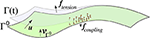Figure 1. Sketch of some objects defined in in sections 2.1, 4 for the abstract framework and the specific cell blebbing model, respectively. The cell membrane Γ(t) is parameterized by u over the initial surface Γ0 which, unlike in this sketch, is closed and of spherical topology. Force densities arising from membrane tension, denoted ftension, point outwards in concave areas (as in the sketch) and inwards in convex areas. Linker molecules (indicated in orange) connect the membrane with the cortex, which is close to the initial surface and not explicitly indicated. They are modeled with a force density that points toward the initial surface when the molecules are stretched. The force vanishes if the linkers break. The pressure fpressure points in the direction of the unit normal ${\nu }_{{\Gamma }^{0}}$ of Γ0, which is the external unit normal of the cell in its initial shape.

### 2.2. Assumptions and Weak Formulation

In order to enable us to approximate (1) with linear finite elements, we split up the fourth order operator by using the curvature

$w=−ΔΓ0u.$

The function ψ : ℝ3×3 → [0, ∞] is assumed to be continuously differentiable with uniformly Lipschitz continuous partial derivative, i.e., denoting with ψ′ this (3 × 3 tensor-valued) partial derivative we assume that there is a constant Cψ > 0 such

This implies that $|{\psi }^{\prime }\left(A\right)|\le {C}_{\psi }|A|+C$ for some constant C > 0. Moreover, we assume that ψ′ is tangential in the following sense:

Note that then ψ′(A)κ = 0 because κ points in the normal direction. The PDE (1) involves the term ${\nabla }_{{\Gamma }^{0}}·{\psi }^{\prime }\left({\nabla }_{{\Gamma }^{0}}u\right)$, and thus by (2)

$∫Γ0(∇Γ0·ψ′(∇Γ0v))·zdσ=-∫Γ0ψ′(∇Γ0v):∇Γ0zdσ$

for sufficiently smooth functions v, z. With a slight abuse of notation we write

The function k: Γ0 × ℝ3 → ℝ3 is assumed to be bounded, measurable with respect to the first argument, and uniformly Lipschitz continuous in the second argument, i.e., there is some constant Ck > 0 such that for all y ∈ Γ0

This implies that |k(y, a)| ≤ Ck|a| + C for some constant C > 0.

The (weak) variational formulation of (1) reads:

Problem 2.1. Find u, wL2(0, T; H10)) with ${\partial }_{t}u\in {L}^{2}\left(0,T;{L}^{2}\left({\Gamma }^{0}\right)\right)$ such that for all ϕ, ηH10) and almost all t ∈ (0, T)

and such that $u\left(·,0\right)={id}_{{\Gamma }^{0}}$.

Remark 2.2. One could consider a function ψ that, like k, depends on the position y on Γ0. For the finite element approximation discussed in the next section, we make use of an approximation kh of k (see around (10)). Something similar could be done for ψ as well. This would result in some additional terms that would need to be estimated using a consistency assumption similar to (10). As the procedure is similar to the one for k we omit the details for conciseness.

## 3. Surface Finite Element Approach

### 3.1. Surface Triangulations and Finite Elements

The membrane Γ0 is approximated by a family of polyhedral surfaces ${\left\{{\Gamma }_{h}^{0}\right\}}_{h}$, each one being of the form

$Γh0=∪E∈𝔗hE⊂ℝ3$

where the E are closed, flat non-degenerate triangles whose pairwise intersection is a complete edge, a single point, or empty. For each E belonging to the set 𝔗h of triangles we denote by h(E) = diam(E) its diameter and then identify $h={\text{max}}_{E\in {𝔗}_{h}}h\left(E\right)$ with the maximal edge length of the whole triangulation. We assume that the vertices of ${\Gamma }_{h}^{0}$ belong to Γ0 so that ${\Gamma }_{h}^{0}$ is a piecewise linear interpolation of Γ0. We also assume that h is small enough so that ${\Gamma }_{h}^{0}$ lies in the thin layer around Γ0 in which the signed distance function d is well-defined. Furthermore, we assume that ${\Gamma }_{h}^{0}$ is the boundary of a domain that approximates Ω(0) and denote the external unit normal, which is defined on the triangles and thus piecewise constant, with ${\nu }_{{\Gamma }_{h}^{0}}$. By ${P}_{h}=I-{\nu }_{{\Gamma }_{h}^{0}}\otimes {\nu }_{{\Gamma }_{h}^{0}}$ we denote the projection to the tangent space in points on ${\Gamma }_{h}^{0}$ where it exists (i.e., in the interiors of the triangles E ∈ 𝔗h). This gives rise to the piecewise (i.e., triangle by triangle) definition of a surface gradient ${\nabla }_{{\Gamma }_{h}^{0}}$ on ${\Gamma }_{h}^{0}$. The same notation ${\nabla }_{{\Gamma }_{h}^{0}}$ is used again for the weak derivative. We write h for the surface area element when integrating functions on ${\Gamma }_{h}^{0}$.

For the error analysis we have to measure the distance of functions such as u on Γ0 to functions such as the finite element solution on ${\Gamma }_{h}^{0}$. For this purpose, we assume that for each ${y}_{h}\in {\Gamma }_{h}^{0}$ there is a unique point y ∈ Γ0 such that

This bijection gives rise to the lift of any function $\eta :{\Gamma }_{h}^{0}\to ℝ$ to Γ0 defined by

Writing μh for the local change of the surface area element, i.e., h = μh, integrals transform as

A straightforward calculation show that in points where both η and η are differentiable

The following two lemmas on the errors due to the approximation of the surface and on the stability of the lift are due to Dziuk , Dziuk and Elliott .

Lemma 3.1. The following estimates hold true for some constant C > 0 independent of h:

Lemma 3.2. Let $\eta :{\Gamma }_{h}^{0}\to ℝ$ with its lifted counterpart η0 → ℝ. Let also E ∈ 𝔗h and ${E}^{\ell }=\left\{y\in {\Gamma }^{0}|{y}_{h}\in E\right\}$ with y as in (7) for a given yh. The following estimates hold true with a constant C > 0 independent of h and the element E:

These inequalities generalize to the whole surfaces by summing over the elements.

The standard finite element space used throughout is

Note that the identic map of ${\Gamma }_{h}^{0}$ belongs to ${S}_{h}^{3}$. Bilinear forms corresponding to m and s are defined for finite element functions ${R}_{h},{Z}_{h}\in {S}_{h}^{3}$ on the triangulation by

and we will also use again the notation ${s}_{h}\left({\psi }^{\prime };{R}_{h},{Z}_{h}\right)={\int }_{{\Gamma }_{h}^{0}}{\psi }^{\prime }\left({\nabla }_{{\Gamma }_{h}^{0}}{R}_{h}\right):{\nabla }_{{\Gamma }_{h}^{0}}{Z}_{h}d{\sigma }_{h}.$ For the discrepancy to the forms on Γ0 we note the following result:

Lemma 3.3. () There is a constant C > 0 independent of h such that for all ${R}_{h},{Z}_{h}\in {S}_{h}^{3}$

where ${r}_{h}={R}_{h}^{\ell }$ and ${z}_{h}={Z}_{h}^{\ell }$.

We define the Ritz projection ${\Pi }_{h}:{H}^{1}\left({\Gamma }^{0}\right)\to {S}_{h}$ by

It's lift is denoted by ${\pi }_{h}\left(\xi \right)={\Pi }_{h}{\left(\xi \right)}^{\ell }$ and has the following approximation properties:

Lemma 3.4. () If ξ ∈ H10) then

and if ξ ∈ H20) then

$‖ξ−πh(ξ)‖L2(Γ0)+h‖∇Γ0(ξ−πh(ξ))‖L2(Γ0)≤Ch2‖ξ‖H2(Γ0)$

where C > 0 is a constant independent of h and ξ.

The projection and the convergence results extend to functions in ${L}_{{H}^{1}}^{2}$ with a pointwise (in time) definition of the projection and with the norms $‖·{‖}_{{H}^{k}}$ replaced by $‖·{‖}_{{L}_{{H}^{k}}^{2}}$, k = 0, 1, 2.

### 3.2. Semi-discrete Problem

In applications, we may only have access to a triangulated surface ${\Gamma }_{h}^{0}$ but not Γ0, for instance, when ${\Gamma }_{h}^{0}$ is computed from image data. In such cases we may also know functions such as k only approximately. For instance, the specific choice (55) of k for our numerical simulations involves the unit normal ${\nu }_{{\Gamma }^{0}}$ and the cortex position uc, both of which may not be known exactly if we only have ${\Gamma }_{h}^{0}$ rather than Γ0. However, we have the approximations ${\nu }_{{\Gamma }_{h}^{0}}$ or ${u}_{c,h}={id}_{{\Gamma }_{h}^{0}}-{l}_{0}{\nu }_{{\Gamma }_{h}^{0}}$ at our disposition. For the analysis of the abstract model we therefore assume that k is approximated by some function (properly, a h family of functions) ${k}_{h}:{\Gamma }_{h}^{0}×{ℝ}^{3}\to {ℝ}^{3}$ that has the same regularity properties as k. In particular, kh is Lipschitz continuous in the second argument with the same Lipschitz constant Ck > 0 independently of h. We define its lift ${k}_{h}^{\ell }:{\Gamma }^{0}×{ℝ}^{3}\to {ℝ}^{3}$ by ${k}_{h}^{\ell }\left(y,a\right)={k}_{h}\left({y}_{h},a\right)$, a ∈ ℝ3, with y and yh related as defined around (7). We assume that kh is an approximation of k in the following sense: There is a constant C > 0 independent of h such that for all a ∈ ℝ3

Problem 3.5. Find ${U}_{h},{W}_{h}\in {C}^{1}\left(0,T;{S}_{h}^{3}\right)×{C}^{0}\left(0,T;{S}_{h}^{3}\right)$ such that for all ${\Phi }_{h},{H}_{h}\in {S}_{h}^{3}$ and all t ∈ (0, T)

and such that ${U}_{h}\left(·,0\right)={id}_{{\Gamma }_{h}^{0}}$.

In the next subsection we will show the following main result:

Theorem 3.6. The semi-discrete problems 3.5 are well-posed for all h > 0 small enough. As h → 0 the lifted solutions $\left({u}_{h},{w}_{h}\right)=\left({U}_{h}^{\ell },{W}_{h}^{\ell }\right)$ converge to some functions (u, w) that uniquely solve the abstract variational problem 2.1 and satisfy

with some C > 0 that depends on data only.

### 3.3. Proof of Theorem 3.6

We generally follow the procedure in Elliott and Ranner . Essential differences consist in the approximation of the data k by kh and the non-linear function ψ′ of the gradient. To deal with the former, the consistency assumption (10) will turn out sufficient, whilst for the latter we will exploit the relations (6) and (12) to show strong convergence of the gradient of the deformation.

Short time existence for (11), (12) is straightforward to show. Estimates are now derived that are, at first, only valid at times of existence but then in the usual way can be used to show existence over the whole time interval by a continuation argument. We therefore state these estimates directly on the whole time interval. We also use the standard notion of C > 0 for a generic constant that depends on the problem data but not on any solution, and which may change from line to line.

Testing with Φh = Uh in (11) and Hh = Wh in (12) and subtracting these identities yields that

Here and in the following we use the Lipschitz continuity of ψ′ and kh, which implies linear growth [see (3), (4) and the comments after]. Choosing Hh = Uh in (12) and applying Young's inequality we see that

for $\stackrel{^}{\epsilon }>0$, and choosing $\stackrel{^}{\epsilon }$ small enough we thus obtain from (14) that

$12ddt‖Uh‖L22+12‖Wh‖L22≤C(‖Uh‖L22+1).$

A Gronwall argument therefore yields the estimate

Testing with Φh = Wh in (11) and Hh = ∂tUh in (12) and then adding these equations yields that

$sh(∂tUh,Uh)+sh(Wh,Wh)=−sh(ψ′;Uh,Wh)−mh(kh(Uh),Wh).$

With Hh = Wh in (12) and using Young's inequality again we get for any small $\stackrel{^}{\epsilon }>0$ that

Using the Lipschitz continuity of ψ′ and kh again we thus can conclude that

Choosing $\stackrel{^}{\epsilon }$ small enough and then applying (15) and a Gronwall argument we obtain the estimate

Taking the time derivative of (12) (note that, using that ∂tUh exists, this equation can be used to show that ∂tWh exists) yields that sh(∂tUh, Hh) = mh(∂tWh, Hh). We test this with Hh = Wh and subtract it from (11) with Φh = ∂tUh to obtain that

Noting that

and using the Lipschitz continuity of kh again we see that

Therefore, with (15) we obtain the estimate

These estimates (15)–(17) are now lifted from ${\Gamma }_{h}^{0}$ to Γ0. We can then apply compactness arguments to deduce the existence of limits (u, w), which we will show to satisfy Problem 2.1. As a first step, the stability estimate (13) will be derived. Using Lemma 3.2 the lifted solutions satisfy the estimates

Hence, there are functions $u\in {L}_{{H}^{1}}^{2}$ with ${\partial }_{t}\in {L}_{{L}^{2}}^{2}$ and $w\in {L}_{{H}^{1}}^{2}$ such that for a subsequence as h → 0

and these limits also satisfy (18) and (19) and, thus, the stability estimate (13).

Let us now show that (u, w) satisfies (6). For any $\eta \in {L}_{{H}^{1}}^{2}$ let Hh = Πh(η) denote its Ritz projection with the lift ηh = πh(η). Then sh(Uh, Hh) = mh(Wh, Hh), whence

By the properties of the Ritz projection (Lemma 3.4) we have that ηh = πh(η) → η in ${L}_{{H}^{1}}^{2}$. Thanks to (20) we thus have that J1 → 0 as h → 0, and similarly J4 → 0 thanks to (21). Lemma 3.3 together with the estimates (16) and (17) ensures that J2 → 0 and J3 → 0 as h → 0. Therefore, (u, w) satisfies the following identity, which implies (6):

Next, we show strong convergence of ${\nabla }_{{\Gamma }^{0}}{u}_{h}$. We note that

Using again Lemma 3.4 we see that πh(u) → u in ${L}_{{H}^{1}}^{2}$, and with (20) this implies that K1 → 0. Regarding the second term we note that thanks to (22) and (12)

As both πh(u) → u and uhu by (21) we see that πh(u) − uh → 0 in ${L}_{{L}^{2}}^{2}$ as h → 0. With whw in the same space we obtain that K21 → 0. From the definition and properties of the Ritz projection it easily follows that $‖{\Pi }_{h}\left(\xi \right){‖}_{{H}^{1}}\le C‖\xi {‖}_{{H}^{1}}$ with some C > 0 independent of h and ξ ∈ H10). The stability estimate (13), which is already proved, and the estimates (15) and (16) therefore yield that $‖{\Pi }_{h}\left(u\right)-{U}_{h}{‖}_{{H}^{1}}$ is uniformly bounded in h. Using (15) and (16) again for Wh and Lemma 3.3 we obtain that K22 → 0 and K23 → 0 as h → 0. This finally shows that

To conclude the proof of Theorem 3.6 we need to show that (u, w) satisfies (5). For any $\varphi \in {L}_{{H}^{1}}^{2}$ let Φh = Πh(ϕ) be its Ritz projection with lift ϕh = πh(ϕ). Then

Thanks to (21) we have that L1 → 0 as h → 0. Lemma 3.4 on the Ritz projection ensures that L2 → 0. It also ensures that Φh is uniformly bounded in h, and with Lemma 3.3 and (17) we obtain that L3 → 0. Altogether

Analogously one can show that

Next, we can write

Thanks to (23) and the Lipschitz continuity of ψ′ we have that ${\psi }^{\prime }\left({\nabla }_{{\Gamma }^{0}}{u}_{h}\right)\to {\psi }^{\prime }\left({\nabla }_{{\Gamma }^{0}}u\right)$ in ${L}_{{L}^{2}}^{2}$ and almost everywhere, whence M1 → 0 as h → 0. For the second term we observe that

thanks to the estimate (16) and Lemma 3.4. In the last term we lift the second integral to Γ0 (recall (8) and (9) for the transformation of the derivative):

We can now apply the Lipschitz continuity of ψ′ and the geometric error estimates in Lemma 3.1 (which imply that $‖{Q}_{h}{‖}_{{L}^{\infty }\left({\Gamma }^{0}\right)}$ is uniformly bounded in h) to obtain that

Using estimate (18) and that also $‖{\varphi }_{h}{‖}_{{L}_{{H}^{1}}^{2}}\le C‖\varphi {‖}_{{L}_{{H}^{1}}^{2}}$ is uniformly bounded (follows from Lemma 3.4) we see that M3 → 0, and we can conclude that

For the last term in (5) we note that

Thanks to (21) and the Lipschitz continuity of k we have that k(uh) → k(u) in ${L}_{{L}^{2}}^{2}$ and almost everywhere, so that N1 → 0 as h → 0. The second term converges to zero thanks to ϕhϕ in ${L}_{{L}^{2}}^{2}$. Regarding N3, we lift the second integral to Γ0:

Using now the consistency (10) of the approximation of k by kh, the Lipschitz continuity of kh, and the geometric error estimates in Lemma (3.1) we obtain that

using estimate (18) and that also $‖{\varphi }_{h}{‖}_{{L}_{{L}^{2}}^{2}}\le C‖\varphi {‖}_{{L}_{{L}^{2}}^{2}}$ is uniformly bounded. Altogether

The convergence results (25), (26), (30), and (34) show that (u, w) satisfies (5), which is the limit of (11) as h → 0.

In the next section we show error estimates. These techniques can also be used to show uniqueness of the solution (u, w) to Problem 2.1. We therefore omit the details for brevity. This concludes the proof of Theorem 3.6.

### 3.4. Error Estimates

Deriving error estimates is possible when assuming higher regularity of the solution, henceforth:

We will derive error estimates on the triangulated surfaces and for this purpose us the bijection (7) to define the inverse lift of the solution (u, w) to ${\Gamma }_{h}^{0}$:

We use the Ritz projection to split the errors into a projection error ρ and a discrete error θ:

Thanks to the regularity assumption (35), the properties of the Ritz projection (Lemma 3.4), and the properties of the lift (Lemma 3.2) error bounds for the projection errors are straightforward:

To estimate the discrete errors let ${\Phi }_{h}\in {S}_{h}^{3}$, test (11) with −Φh and test (5) with −ϕh, which is the lift of Φh. We get that

Now we add the terms mh(∂tΠh(u), Φh), shh(w), Φh), ${s}_{h}\left({\psi }^{\prime };{\Pi }_{h}\left(u\right),{\Phi }_{h}\right)$, and mh(khh(u)), Φh) on both sides. Using that shh(w), Φh) = s(w, ϕh) by the definition of the Ritz projection this yields that

Proceeding similarly with (6) and (12) for any ${H}_{h}\in {S}_{h}^{3}$ with lift ηh we obtain that

The error terms satisfy the following estimates:

Lemma 3.7. There is some C > 0 independent of h (sufficiently small) such that for all ${\Phi }_{h},{H}_{h}\in {S}_{h}^{3}$

Proof: To show the estimates, we will frequently apply Lemma 3.3 on the approximation of the bilinear forms, Lemma 3.2 on the stability of the lift, and Lemma 3.4 on the Ritz projection without explicitly pointing it out for conciseness.

The Ritz projection commutes with the time derivative thanks to the regularity of u. Therefore

The term ${\stackrel{~}{L}}_{3}$ is similar to L3 in (24) but without the time integral and with πh(∂tu) instead of uh and thus can also be estimated similarly:

Furthermore,

which altogether yields (40).

We can also split up Eψ:

The first term ${\stackrel{~}{M}}_{3}$ is similar to the term M3 in (27), without the time integral and with πh(u) instead of uh. Following the lines of (28) and (29) we obtain that

Using that ψ′ is Lipschitz, the other term is estimated as

which together shows (41).

For the third estimate we use the splitting

Noting and exploiting the similarity of Ñ3 with N3 in (27) we proceed as in (32) and (33) to obtain that

Furthermore,

$|N˜1|≤Ck‖πh(u)−u‖L2(Γ0)‖ϕh‖L2(Γ0)≤Ch2‖u‖H2(Γ0)‖Φh‖L2(Γh0),$

which finally yields the estimate (42).

The last estimate (43) can be proved analogously to (40). This concludes the proof of Lemma 3.7.

With these estimates we can derive the following estimates for the error:

Theorem 3.8. Assume that (u, w) solves Problem 2.1 and satisfies $u,{\partial }_{t}u,w\in {L}_{{H}^{2}\left({\Gamma }^{0}\right)}^{2}$. For all sufficiently small h the solution (Uh, Wh) of Problem 3.5 satisfies

with a constant C > 0 independent of h.

Proof: We proceed as for deriving (15) but start with (38), where we test with ${\Phi }_{h}={\theta }^{\left(u\right)}$, and with (39), where we choose ${H}_{h}={\theta }^{\left(w\right)}$. Taking the difference we obtain that

In Lemma 3.7 we absorb the norm of u into C to obtain that

$|Et(θ(u))|≤Ch4+12‖θ(u)‖L2(Γh0)2,|Eψ(θ(u))|≤Ch2+12‖∇Γh0θ(u)‖L2(Γh0)2,$

and similarly for the other two errors. Using that ψ′ and kh are Lipschitz we then get that

Substituting ${H}_{h}={\theta }^{\left(u\right)}$ in (39) gives for any $\stackrel{^}{\epsilon }>0$ that

We can thus estimate the terms involving $‖{\nabla }_{{\Gamma }_{h}^{0}}{\theta }^{\left(u\right)}{‖}_{{L}^{2}\left({\Gamma }_{h}^{0}\right)}^{2}$ on the right-hand-side of (44) by terms involving $\stackrel{^}{\epsilon }‖{\theta }^{\left(w\right)}{‖}_{{L}^{2}\left({\Gamma }_{h}^{0}\right)}^{2}$. Choosing now $\stackrel{^}{\epsilon }>0$ small enough, these terms involving $‖{\theta }^{\left(w\right)}{‖}_{{L}^{2}\left({\Gamma }_{h}^{0}\right)}^{2}$ can then be absorbed in the left-hand-side to that altogether

$ddt‖θ(u)‖L2(Γh0)2+‖θ(w)‖L2(Γh0)2≤C‖θ(u)‖L2(Γh0)2+Ch2.$

By standard interpolation theory (recall that the identic map of the triangulated surface ${\Gamma }_{h}^{0}$ linearly interpolates the identic map of Γ0) the initial error satisfies

Applying Gronwall therefore yields that

$‖θ(u)‖LL2(Γh0)∞2+‖θ(w)‖LL2(Γh0)22≤Ch2.$

From (45) we now see that also

$‖∇Γh0θ(u)‖LL2(Γh0)22≤Ch2.$

Together with (36) and (37) these two estimates conclude the proof of Theorem 3.8.

## 4. Continuum Model for the Onset of Blebbing

### 4.1. Force Contributions

Mathematical models that aim to provide insight into the mechanisms that control cell blebbing require an approach to describe the evolving geometry and have to account for various force contributions acting on the plasma membrane. Based on previous ideas  we postulate that a force balance of the form

governs the cell membrane's shape. The contributions are force densities on the cell membrane and are modeled in a form such that a surface partial differential equation of the form (1) is obtained. Figure 1 gives an idea of the setup. We specifically aim for generalizing the model in Tyson et al.  and Collier et al.  for curves in 2D to surfaces in 3D but also put the force contributions into the wider literature context.

Pressure: Building up internal pressure, for instance, by actin-myosin contraction in the cortex, is essential for blebbing (see Charras et al.  and references). We write the corresponding force density as

where $V\left(u\right)=\text{max}\left\{{\int }_{{\Gamma }^{0}}\frac{1}{3}u·{\nu }_{{\Gamma }^{0}}d\sigma ,0\right\}$ is an approximation of the volume of Ω and p0 is a pressure coefficient so that p0/|V(u)| is the pressure difference between interior and exterior of the cell. This simple assumption of a constant (in space) pressure difference should be sufficient to study the influence of the initial shape on the blebbing propensity. However, for the dynamics of blebs the pressure distribution will be of importance and requires to model the ambient fluid as in Strychalski and Guy  and Fang et al.  and probably also the actin-myosin biochemistry.

Coupling between membrane and cortex: Forces arise due to molecules connecting the membrane with the actin cortex, and when the membrane detaches during the blebbing process these linkers break. Figure 1 gives an impression of such a force (denoted by fcoupling). An actin scar is left behind the bleb [4, 7] that disintegrates in the longer run, and the cortex reassembles close to the new membrane position. However, as we are interested in short times and the onset of blebbing we assume the cortex to be stationary and positioned a small distance l0 away from the initial membrane. Connection points of linkers in the cortex are given by ${u}_{c}={id}_{{\Gamma }^{0}}-{l}_{0}{\nu }_{{\Gamma }^{0}}$. The linker molecules can be modeled as the density of simple springs with parameter kl and assumed to be initially at rest. In a continuum setting this can be modeled with an energy density ${e}_{coupling}=\frac{{k}_{l}}{2}{\left(|u-{u}_{c}|-{l}_{0}\right)}^{2}$ as long as they are intact. As a critical length uB is exceeded they break. Moreover, when they get closer than a distance uR to the cortex then the repulsion force is increased to prevent any intersection. A model for the force density thus reads

with

with some constant kL > 0 and the Heaviside function H(r) = 1 if r ≥ 0 and H(r) = 0 otherwise. The assumption of a constant kl, which corresponds to a homogeneous linker, is a simplification. The cell usually is able to control the density and, thus, the local effective linker strength by its biochemistry . We briefly report on a way to account for membrane-bound biochemistry in section 5.5.

Tension: Lateral tension in curved membranes leads to net forces in normal direction that, in the case of isotropic materials, are proportional to the curvature. The distribution of tension is of relevance in moving cells . It has been noted in Tyson et al.  and further investigated in Collier et al.  that the propensity of blebs in concave regions of the cell membrane is higher. In such areas, the force due to the membrane tension points outwards (Figure 1 gives an impression, the force is denoted by ftension) and then adds to the pressure whilst in convex regions it opposes the pressure. We consider an energy density of the form

where kψ > 0 and x0 ∈ [0, 1] are parameters. Note that $|{\nabla }_{{\Gamma }^{0}}u\left(·,0\right)|=|{\nabla }_{{\Gamma }^{0}}{id}_{{\Gamma }^{0}}|=|P|=\sqrt{2}$ so that in the case x0 = 1 the membrane initially is at rest.

If Γ0 was a curve in 2D then we would obtain the model in Tyson et al.  and Collier et al. , which is motivated by chains of linear springs with spring constant kψ and resting length x0. More sophisticated elastic energies for membranes than (50) can be derived from discrete models for meshes formed by springs [37, 38] and then usually also account for resistance to bending (discussed further below around (52)). Let us also note that biomembranes, the basic component of cell membranes, rupture when stretched beyond a few percentages. Whilst this seems satisfied by the small deformations during the onset of blebbing that we study, the area increase during full bleb formation can be more significant and require the supply of membrane area , which then is likely to affect the tension, too.

The (tension) force density is given by minus the variation of our energy (50),

Regularization: The membrane resists bending, though much less than stretching. The corresponding elastic energy may be modeled as in Helfrich  and Woolley et al. , see  for a discussion of minimal approaches. The impact of the bending force on the blebbing site selection and its shape has been found to be significantly smaller than that of the tension . However, we suspect that it is of relevance in the area where the bleb is connected with the cell as there the curvature and its derivative can be high. Therefore, we want methodology and software capable of addressing this aspect for future studies. We here choose a simple linear bending model that may be considered as a regularization with the energy density

$ereg=kb2|ΔΓ0u|2$

where kb is a (small) bending resistance coefficient. Minus its variation yields the regularization force density

Viscous drag: Blebbing approaches often explicitly account for the fluid in the interior and exterior of the cell, which then is assumed Newtonian and viscous [6, 7, 9]. Our focus is on the onset of blebbing rather than on the time evolution and the growth of blebs. We therefore only postulate a viscous drag force density that opposes any membranes movement:

$fdrag=−ω∂tu,$

where ω is an effective material parameter related to the viscosity of the ambient fluid. Though this approach might yield a good approximation to the dynamics, at least at short time scales, it should rather be considered as another regularization term and a mean to decrease membrane energy in a controlled way. It may also enable us to compute bleb shapes, noting that the drag force is zero if the membrane is at rest, i.e., if ∂tu = 0. To address questions such as the origin of the fluid in the bleb (from outside of the cell via pores in the membrane  or from inside through the cortex ) our approach will be insufficient, of course.

With these choices, the force balance (46) yields the PDE.

### 4.2. Non-dimensionalization and Regularization

The PDE (53) has been non-dimensionalized by choosing a length scale U and by using kψ as an energy density scale. Choosing the time scale $\Theta ={U}^{2}\omega /{k}_{\psi }$ then eliminates the viscosity parameter ω. We furthermore define the non-dimensional parameters ${\lambda }_{b}={k}_{b}/\left({U}^{2}{k}_{\psi }\right)$, ${\lambda }_{l}={k}_{l}{U}^{2}/{k}_{\psi }$, and ${\lambda }_{p}={p}_{0}/\left({U}^{2}{k}_{\psi }\right)$ and note that x0 and kL are non-dimensional already. Writing again Γ0, u, uc, uB, uR, l0, and V(u) for the respective non-dimensional objects, Equation (53) in non-dimensional form and rearranged reads

Note that the model in Tyson et al.  and Collier et al.  is obtained by reducing the dimension of this Equation (54) (i.e., Γ0 is a curve in 2D). The curve then is parameterized by arc-length, and their computational model is obtained by using standard finite difference techniques.

Equation (54) can be cast in the form (1) (without loss of generality we can assume that λb = 1 as we may divide by λb, rescale in time, and absorb the 1/λb term into k and ψ defined below) by defining

and

Note neither k nor ψ′ satisfy the Lipschitz continuity conditions in section 2.2. We still used them for the simulations in section 5 but also have redone some simulations with regularized versions of k and ψ′. To ensure that the assumptions in section 2.2 are satisfied, smoothing the Heaviside function and ensuring that the denominators do not degenerate is sufficient. With a small parameter ε > 0 the choice

$λcoupling,ε(y)=λl(1+kL1+exp(2(y−uR)/ε))11+exp(2(y−uB)/ε)$

and then

satisfies the assumptions around (4). The approximation

with

$Vh(Uh)=max{∫Γh013Uh·νΓh0dσh,0}$

satisfies the consistency assumption (10). Similarly, the choice

$ψ(∇Γ0u)=12(|∇Γ0u|2+ε−2x0)2$

so that

satisfies the assumptions around (3).

## 5. Software and Simulations

### 5.1. Time Discretisation

We performed some numerical simulations for (54) to illustrate the capability of the theoretical framework that has been presented and analyzed. The variational form with operator splitting in Problem 2.1 is discretised in time with a simple semi-implicit first order scheme as follows: We split the time interval [0, T] into M ∈ ℕ equal parts of size τ = T/M, denote the time steps with t(m) = , and write f(m) = f(t(m)) for any time dependent fields or functions.

Problem 5.1. Given ${\Gamma }_{h}^{0}$, ${S}_{h}^{3}\ni {u}_{c,h}\approx {u}_{c}$, and parameters λb, λl, λp, l0, uB, kL, uR, for m = 0, …, M − 1 find $\left({{U}_{h}}^{\left(m+1\right)},{{W}_{h}}^{\left(m+1\right)}\right)\in {S}_{h}^{3}×{S}_{h}^{3}$ such that for all $\left({\Phi }_{h},{H}_{h}\right)\in {S}_{h}^{3}×{S}_{h}^{3}$

with

$λcoupling(m)=λl(1+kLH(uR−|Uh(m)−uc,h|))H(uB−|Uh(m)−uc,h|).$

### 5.2. Implementation

We have solved the above problem using the Python bindings from the DUNE-FEM module , which is based on the Distributed and Unified Numerics Environment (DUNE) . DUNE is an open source C++ environment that uses a static polymorphic interfaces to describe grid based numerical schemes. The package provides a large number of realizations of these interfaces including many finite element spaces on structured and unstructured grids. This approach allows for the efficient and flexible simulation of a large variety of mathematical models based on partial differential equations.

The Python bindings described in Dedner and Nolte  simplify the rapid prototyping of new schemes and models, while maintaining the efficiency and flexibility of the DUNE framework. This is achieved by using the domain specific language UFL  to describe the mathematical model and implementing the high level program control within Python. All computationally critical parts of the simulation are carried out in C++ using just in time compilation of the required DUNE components. Consequently, the assembly of the bilinear forms and solving of the linear and non-linear problems is implemented in C++ while the time loop and the input and output of data is carried out using the Python scripting language. More information on the concepts can be found in Dedner et al. .

Meshes can be provided using a GMsh file or, as done for this work, by using the internal Dune Grid Format (DGF). All simulations reported on in this paper were performed using a first order Lagrange space over a simplicial, locally adaptive, distributed grid, which can be used for both bulk and surface domains . Bindings for a number of different solver packages are available through DUNE-FEM including the iterative solvers from DUNE-ISTL  (used for this work), direct solvers from the SuiteSparse package , and a number of solvers and preconditioners from the PetSc package . The simulation results were exported using VTK and visualized using ParaView .

In the following we show how to setup the grid and how some parts of the mathematical model are defined within UFL. The full code needed to perform the simulations shown in this paper is available (see the Data Availability Statement).

The first listing shows how to read in a grid for a cell obtained from experimental data that was used for the simulations in section 5.4:

from dune.alugrid import aluSimplexGrid

from dune.fem.space import lagrange

surfaceGrid = aluSimplexGrid(“cell.dgf”,

dimgrid=2, dimworld=3)

solutionSpace = lagrange(surfaceGrid,

dimRange=3, order=1, storage=“istl”)

# a vector-valued finite element function for the position,

# initialized with the vertex positions of the initial grid

position  = solutionSpace.interpolate

(lambda x: x, name=“position”)

# another finite element function, later on used to store the previous time step

position_n = position.copy()

The following snippet demonstrates how the bending terms and tension terms are defined using UFL. The remaining terms, e.g., for the pressure and the linker-molecules, are defined in a very similar way:

from ufl import TrialFunction,

# test and trial function used to define the bilinear forms

u   = TrialFunction(solutionSpace)

phi = TestFunction(solutionSpace)

w   = TrialFunction(solutionSpace)

eta = TestFunction(solutionSpace)

# the bending terms using operator splitting

op_split_curv_im = -inner(w, eta)

# the tension terms

tension_ex = sqrt(2.0) * x_0 *

In each time step a saddle point problem is solved using a Uzawa-type algorithm where a CG method is used to invert the Schur complement as described, for example, in Braess . The main algorithm is implemented in Python calling C++ routines to compute the matrix-vector operations and to solve the inner problem. The time loop with the solver is fairly long and doesn't seem worthwhile listing here but, as stated above already, the whole code is publicly available.

A number of tests have been performed for problems with known solutions (u, w) to validate the convergence (rates) of Theorems 3.6 and 3.8. Recall that the choices of the tension term ψ (56), and the coupling term k (55), in the specific model (54) do not satisfy the requirements of the analysis. However, in our simulations, the denominators in these terms did not become very small. Comparative simulations with the regularized choices (58) and (57) with ε = 10−5 did not reveal any essential difference. More details on computations to support the theoretical results and validation of the code can be found in Nixon . For conciseness, we don't report on these here but focus on an investigation of the parameter space instead.

### 5.3. Brief Parameter Study

One question of interest has been whether surface tension and pressure are sufficient to initiate blebbing without any weakening of the cortex, as found in Collier et al.  in 2D. We also further study the parameter space but remark that the simulation results are at a qualitative level. An in-depth discussion involving quantitative information is beyond the scope of this article and left for future investigations. The parameters are steered into the software at the high-level python interface which, in principle, can be conveniently automated at that level for more substantial parameter studies.

We consider an initial shape Γ0 obtained by deforming a sphere of radius one by (all lengths in 10−6m)

with $r=\sqrt{{\left(4{y}_{1}\right)}^{2}+{\left(4{y}_{2}\right)}^{2}}$. This yields a shape similar to a discocyte (or red blood cell, see Figure 2) with a volume of about $V\left({id}_{{\Gamma }^{0}}\right)\approx 1.5×1{0}^{-16}{m}^{3}$ and a largest distance of 4.0 × 10−6m from the center. Let us briefly motivate this choice for the initial shape. In the concave parts the force due to the membrane tension points outwards and in the convex parts it points inwards. When the cell membrane moves away from the cortex then the coupling force, which is due to stretched linker molecules as long as these are not broken, points inwards. Therefore, if pressure and tension are able to overcome the coupling force and initialize a bleb we will expect this to happen first (and possibly only) in the concave parts of the initial shape.

FIGURE 2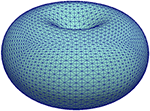Figure 2. Illustration of the initial shape used for the simulation in section 5.3 by means of its mesh ${\Gamma }_{h}^{0}$. For better visibility of the triangles, only ten bisections were performed resulting in a mesh with 20480 vertices. A finer mesh with 196608 vertices was used for the computations.

Parameters for the various force densities vary in the literature, not least due to differing cell types and differences in the models. For the tension coefficient we chose ${k}_{\psi }=1.5×1{0}^{-5}N/m$ (ranges from 2.0 × 10−6N/m  to 1.0 × 10−4N/m ), for the bending coefficient ${k}_{b}=7.5×1{0}^{-20}Nm$ (between 1.0 × 10−20Nm  and 2.0 × 10−19Nm ), and for the linker spring coefficient ${k}_{l}=2.7×1{0}^{6}N/{m}^{3}$ (close to 2.67 × 106N/m3 in Strychalski and Guy ). The parameters x0 = 0.95, ${l}_{0}=4.0×1{0}^{-8}m$, and ${u}_{B}=5.6×1{0}^{-8}m$ were chosen as in Collier et al. . The parameters ${u}_{R}=7.5×1{0}^{-9}m$ and kL = 500.0 were chosen ad hoc but repeating some simulations with kL = 0 (particularly those with higher tension so that the membrane got closer to the cortex) didn't reveal any visual difference. As discussed earlier around (47), pressure inside of the cell has to be higher than outside of it to initiate blebbing. In the literature we find values between 10Pa  and 81Pa . We found that a value of p0/|V(u(0))| ≈ 2.25Pa was already sufficient in our simulations to break the linker molecules and, thus, to generate a bleb site. With a length scale of U = 1.0 × 10−6m the set of non-dimensional parameters is given in Table 1 and was used for simulations unless stated otherwise.

TABLE 1

A triangulation ${\Gamma }_{h}^{0}$ is obtained by starting with a cube with vertices on the unit-sphere, then diagonally cutting the square faces into triangles, and then bisecting all triangles 14 times such that the longest edge is halved. New vertices are projected to the unit-sphere after each refinement step. After, the above map (61) is applied to the 196608 vertices. Figure 2 gives an impression of a mesh thus obtained but with ten refinements only. The time step size was set to τ = 0.0025. As mentioned, the model serves to study the onset of blebbing and we therefore ended the simulation at T = 2. At that time the final shapes usually weren't at rest yet but the deformations were sufficient to break linker molecules and thus generate a blebbing site.

Figure 3 gives an overview of some shapes at the final time for the data set in Table 1 and some variants (see Figure caption for details). The initial shape Γ0 is axisymmetric around its central axis. The initial shape ${\Gamma }_{h}^{0}$ for the computations is not. However, on visual inspection, also the computed shapes look approximately axisymmetric. For this reasons, we compare cuts through the centers for more insight. Figure 4 displays the slices through the initial and the final shape that is visible in Figure 3A. The color code from Figure 3 is used again so that parts of the membrane with broken linkers are colored red. Differences are predominant in the concave part of the initial shape, where the membrane has moved outwards and detached. The tension force in such concave parts points outwards and, together with the pressure, initiates a bleb without requiring any weakening of the cortex. This simulation thus supports the finding in Collier et al. .

FIGURE 3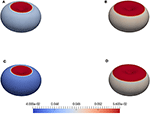Figure 3. Final shapes for computations with the initial shape in Figure 2. The color scheme indicates the distance of the membrane to the cortex |Uhuh, c|. Values below the resting length l0 = 0.04 are highlighted in blue and values above the critical length of breaking uB = 0.056 in red, whilst values in between are shaded as indicated on the bar. The parameters in Table 1 lead to the upper left shape (A). For (B), the linker strength was reduced by setting λl = 12. For (C), the tension was increased by setting x0 = 0.85. For (D), the pressure was increased by setting p0 = 30.

FIGURE 4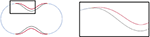Figure 4. Slices through the central axis of shapes obtained in simulations that we report on in section 5.3. A magnified image of the black box is presented on the right. The initial (dark gray) and the final shape (red/light blue) for the computation with the data from Table 1 are displayed. The color code is as in Figure 3. Strongly deformed parts of the membrane, where the linkers are broken, are red and predominant in the concave part of the initial shape.

In Figure 5, we compare the final shapes for different parameters of the linker strength λl, more precisely, slices of the shapes in Figures 3A,B. Note that the color code is different (see caption of Figure 5). The deformation isn't much stronger as, once the membrane is detached, the linker term doesn't influence the evolution any further. But a weaker linker strength λl and, thus, less resistance to breaking leads to a wider bleb site.

FIGURE 5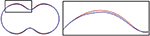Figure 5. Slices through the central axis of shapes obtained in simulations that we report on in section 5.3. A magnified image of the black box is presented on the right. The final shapes for two computations are displayed. One for the standard parameters in Table 1 (blue), and one with a smaller linker strength parameter, namely λl = 12 (red). In the latter case, the forces that keep the membrane attached to the cortex are smaller (see Equation (50), (51), λl corresponds to kl in the dimensional model). The area where the membrane has detached from the cortex is bigger, so the blebbing region is wider.

A smaller resting length parameter x0 increases the surface tension, which leads to a faster evolution and a stronger final deformation. This is visible in Figure 6 where we compare the slices of the shapes in Figures 3A,C, and the (red) curve for the smaller x0 indicates that the membrane has moved further away from the initial shape.

FIGURE 6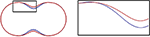Figure 6. Slices through the central axis of shapes obtained in simulations that we report on in section 5.3. A magnified image of the black box is presented on the right. The final shapes for two computations are displayed. One for the standard parameters in Table 1 (blue), and one with x0 = 0.85 (red). The latter effectively increases the membrane tension but also reduces the area at which the membrane is at rest (see Equations (52), (53) and in between). In the concave region of the initial surface the membrane thus moves farther and its area shrinks further than before.

The impact of a higher pressure is illustrated in Figure 7 where slices through the shapes in Figure 3A (blue) and Figure 3D (red) are overlayed. The effect resembles a bit that of a smaller linker strength in that the deformation isn't much different and in that the bleb site is much bigger. The pressure term doesn't break down after detachment and continues to push outwards, though, so that the membrane has moved a bit further throughout the bleb site.

FIGURE 7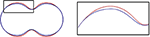Figure 7. Slices through the central axis of shapes obtained in simulations that we report on in section 5.3. A magnified image of the black box is presented on the right. The final shapes for two computations are displayed. One for the standard parameters in Table 1 (blue), and one with a higher pressure parameter, namely p0 = 30 (red). In the latter case, the cell membrane is further pushed outside, and the area where the membrane has detached from the cortex is wider.

### 5.4. Application to Experimental Data

Apart from given, “in-vitro” geometries and their influence on blebbing, users may also be interested in studying the effect of “in-vivo” geometries that are obtained from experimental data. The image postprocessing outlined in Du et al.  enables users to extract triangulated surfaces representing the cell membrane from 3D images of cells, which then can be steered into the software framework. This was done with data of a Dictyostelium cell (also used in Du et al. ) moving by actin-driven pseudo-pods without any blebbing. However, the purpose is again to showcase the capability of the software framework rather than to extract any quantitative information, which is left for future investigations.

We used the non-dimensional parameters in Table 2, T = 20, and τ = 0.02. Figure 8, left, shows the triangulated surface ${\Gamma }_{h}^{0}$ that has been obtained from the image data. On the right of Figure 8 the final shape is displayed where the same color code as in Figure 3 for the deformation strength is used. As in the simulations before we observe that blebs form in concave regions. We also see some deformations at the sides where small protrusions become quite spiky. Both tension and resistance to bending are expected to prevent any singularities to occur, however the geometry seems under-resolved by the mesh in these areas.

TABLE 2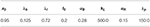Table 2. Non-dimensional parameters for the simulation with an initial surface obtained from image data, which we report on in section 5.4.

FIGURE 8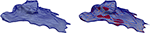Figure 8. Application of the scheme in Problem 5.1 to a cell surface obtained from image data with the parameters from Table 2. The color scheme is as in Figure 3. See section 5.4 for further details.

### 5.5. Extensibility

Due to the use of the Python scripting language for the high level control of the simulation, it is fairly easy to extend our mathematical model to include additional effects. So for example we can conveniently add surface reaction-diffusion equations to model some biochemistry occurring on the membrane, i.e., adding a system of the general form

for some reactants c: Γ0 → ℝr, where the function R:ℝr → ℝr describes the reactions. The evolution of c can depend on the evolution of the membrane, i.e., both the diffusion tensor D and the source and sink term R may depend on the deformation u. The reactants c may just be passive in the sense that they do not influence the evolution of the membrane but, in general, they will enter the evolution equation for the surface deformation. For example, they may change some material parameters and, thus, the forces acting on the membrane.

As a proof of concept, we investigate the effect of adding a chemical signal (scalar, c maps to ℝ) to the model to contain the spread of the bleb. In the current model a bleb will, in general, not remain localized but the membrane will detach from large parts of the cortex. In experiments, bleb protrusion stops, forming a fairly spherical cap attached to the part where membrane and cortex still seem connected . To reproduce this phenomenon at least qualitatively, we add a scalar signal c that is initially zero but is subsequently produced where the linkers are broken, and it then diffuses along the membrane. In regions where the linker molecules are still intact the signal leads to an increase of the linker strength. This can prevent further detachment of the cortex and effectively stop any further protrusion of the bleb. We can localize this effect further by making the diffusion tensor D in the reaction-diffusion equation for the signal c depend on the cortex detachment from the membrane. More precisely, we may assume a large diffusion coefficient in regions where |uuc| > uB and a small diffusion coefficient elsewhere.

The variational form of the equation for the signal reads

for test functions z with initial condition c(·, 0) = 0 and augments Problem 2.1. Here, Dc > 0 is a diffusivity parameter and χ(u) = H(|uuc| − uB) (recall that H denotes the Heaviside function) is the characteristic function of the region where the cortex is detached. In the diffusion term, df is a reduction factor. If df = 1 then the diffusion is a uniform constant Dc while if df < 1 then the diffusion is reduced by this factor in regions where the cortex is still attached. The signal is produced at a rate rc > 0 as soon as the cortex detaches up to a maximum value of lc. We discretise (63) in space using the surface finite element method introduced in section 3. In time we treat the diffusion term implicitly and the reaction term explicitly, resulting in a linear problem in each time step. The model for the signal evolution can be conveniently added to the code with a couple of lines:

from ufl import conditional

space_c  = lagrange(surfaceGrid,

dimRange=1, order=polOrder, storage=“istl”)

signal   = space_c.interpolate(space_c.

dimRange*, name=“c”)

signal_n = signal.copy()

detached = conditional(norm(corDistVec)  <  u_B, 0, 1)

D              = D_c * (detached+(1-detached)*d_f)

signalTerms_ex =

r_c * detached * (l_c-signal_n)*z

signalModel    =

(inner(c,z)+tau * signalTerms_im) * dx == \

(inner(signal_n,z)-tau*signalTerms_ex) * dx

As stated above, we assume that the signal is not passive but influences the linker strength, i.e., λl in (55) is replaced by a c dependent function. We want the linker strength to increase from the original value λl to some value λL > λl when c increases from some value cb > 0 to some value cB > cb. For this purpose we define

$l(c)=λl+ξ(c)(λL−λl)$

where ξ is a piecewise defined monotone C1 weight function with ξ(c) = 0 for c < cb and ξ(c) = 1 for c > cB:

cubic = lambda x:

(x-c_b)**2 * (3*c_B-c_b - 2*x) / (c_B-c_b)**3

xi    = lambda x: conditional(x < c_b,0,

conditional(x>c_B,1,cubic(x)))

l     = l_l + (l_L-l_l)*xi(signal_n)

Note that we treat the impact of the signal on the surface evolution explicitly in time to simplify the presentation. Consequently, we can solve for the new membrane deformation and the new signal concentration in separate steps within the time loop. Details are available in the published code (see the Data Availability Statement). In summary, the signal evolution and its interaction with the surface evolution is characterized by seven additional constant parameters, Dc, lc, rc, df for the signal and λL, cb, cB for the surface.

We test the effect of the signal on the evolution of blebs with an artificial surface geometry but with less symmetry than in section 5.3. Figure 9, left, gives an impression of Γ0. In particular, the surface is not rotationally symmetric. Non-dimensional parameters that remain fixed for all simulations that we report on further below are given on the left of Table 3. They coincide with the parameters used in section 5.3 (see Table 1) except for a smaller x0 (this increases the tension force) and a larger λp (this increases the pressure difference). All the simulations reported on in this subsection were carried out on a triangulated surface with around 40 000 elements with a time step of 0.001. To reach time t = 5 required about 1h of computing time on a single core of an Intel Xeon E5-2667 3.20GHz processor.

FIGURE 9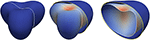Figure 9. Surface evolution with parameters given by Table 3 for the extended model in section 5.5 but with rc = 0 so that the signal c remains zero. The left figure shows the initial surface. The white curve indicates a plane along which we cut the shapes for visualization purposes in Figures 10,11. In the middle and on the right, both the initial surface and the deformed surface are displayed at the final time T = 5. The initial surface is fully displayed with the white line in the middle and cut on the right to illustrate the cutting procedure. The coloring of the original surface indicates the magnitude of the deformation as in Figure 3.

TABLE 3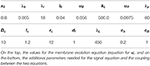Table 3. Non-dimensional parameters for the numerical simulations discussed in section 5.5.

We first checked the case rc = 0, for which c = 0 solves (62), i.e., there is no signal. Figure 9, middle and right, displays the surface at the final time T = 5. We spot that the cortex has detached from the membrane everywhere. We will show now that this can be prevented from happening by accounting for a signal and a strengthening of the linker terms.

We next consider the case df = 1 so that the diffusion parameter Dc is constant. The additional parameters required for the signal and the coupling are given on the right of Table 3. The evolution of the surface with signal is shown in Figure 10. We see the signal being generated where the deformation is strong, i.e., where membrane moves away from the initial shape and thus from the cortex. However, at time t = 5 the membrane is still close to the initial shape in regions away from the concave parts. Comparing this with the simulation without the signal (see Figure 9, right) it is clearly visible that the increase of the linker strength due to the diffusing signal leads to a localization of the blebs. We also see in Figure 10 that the shape is not stationary at time t = 5 but the deformation continues to grow, which we now want to prevent.

FIGURE 10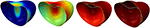Figure 10. Surface evolution at time t = 0.25, 1.25, 2.5, 10 for the model in section 5.5 with the parameters given in Table 3. The diffusion of the signal is a uniform constant. The color from blue via green and yellow to red corresponds to the signal strength: Dark red indicates c > cB, i.e., regions where the linkers (if not broken yet) are maximally strengthened by the signal c, dark blue indicates c < cb and thus regions where the linker strength is not altered by c.

We reduce the diffusion coefficient in regions where the linkers are not broken by setting df = 0.01 whilst keeping the other parameters as in the previous simulation. The expectation is that the strengthening of the linkers is more focused on the boundary of the bleb than before and increases there faster as the signal is not transported away by the diffusion. Thus, also the linker strength should increase faster at the boundary of the bleb. The simulation result is demonstrated in Figure 11. Now, the simulation reaches a stationary state at time t ≈ 2.5 with a small bleb that has developed in the concave region at the top of the surface. At the beginning of the simulation, secondary protrusions also start to develop at the three saddle-shaped sides of the surface. Subsequently, the resulting production of the signal c leads to a strengthening of the linkers, which then pull the cortex back so that the protrusion disappears again.

FIGURE 11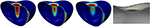Figure 11. The left three figures show the surface evolution at times t = 0.25, 1.25, 5 for the model in section 5.5 with the parameters given in Table 3 but with df = 0.01, i.e., a reduction of the diffusivity of the signal to 1% of its original value in the region where the linkers are not broken. The color corresponds to the signal strength and is the same as in Figure 10. The shape at the final time T = 5 is a stationary state. The figure on the very right displays slices through the shapes at the final time T = 5 for two different grid resolutions and time steps. The yellow curve is the stationary state corresponding to the left three figures, i.e., using around 40000 element and a time step of 0.001. The green curve corresponds to the final state on 160000 elements and with a time step of 0.0005. As before, the white curve shows the initial surface. The final deformed surface with the coarser triangulation also serves to give an impression of the grid resolution compared to the size of the developed bleb.

## 6. Conclusion

A general modeling framework for the onset of blebbing has been presented and analyzed. It is formulated in terms of partial differential equations on the initial membrane, which is considered as a hypersurface. Various forces acting on the plasma membrane due to its elastic properties, linker molecules coupling it to the cell cortex, and pressure difference across the membrane are accounted for. Fluid flow within and outside of the cell is essentially neglected modulo a drag force but may be considered in future studies.

The general framework is particularly flexible with regards to membrane tension and the coupling forces. A convergence analysis of a surface finite element discretisation shows its robustness to model alterations within not too restrictive limits. There are some open questions with regards to the discretisation in time, and as blebs are local events, spatial mesh adaptivity may be beneficial.

Software for a specific instance of the general model is provided and has been used to perform some numerical simulations. A convenient high-level interface in Python allows for directly implementing the model in its variational form and solving it by an efficient software back-end. Standard software usually does not provide functionality for numerically solving problems on moving domains or hypersurfaces in 3D out of the box but requires a substantial amount of coding. We hope that our approach will address this issue and simplify the implementation of such moving boundary problems. Future work on the software will include improvements of the efficiency of the implementation, by investigating, for example, the use of well-established techniques like local grid adaptivity and parallelization (which are available through the grid manager used for the simulations shown here ) as well as incorporating more sophisticated preconditioning methods in the linear solver implementation. This will be an important step toward extending the model to include, for example, fluid flow inside and outside of the cell.

## Data Availability Statement

The Python scripts used to obtain the results reported on here are available in a git repository hosted on the DUNE gitlab server: https://gitlab.dune-project.org/bjorn.stinner/sfem_blebs.

The main scripts are blebbing_artgeom.py, blebbing_imgdata.py, and blebbing_signal.py used for the results from sections 5.3, 5.4, and 5.5, respectively. The setup of the model, the time loop, and the solver used are contained in blebbing_compute.py. Some auxiliary functions can be found in blebbing_tools.py.

The experiments reported on in this paper, can be reproduced using the DUNE-FEM docker container. A script “startdune.sh” is available in the git repository to download and start the container. This requires the “docker” software to be available on the system. It can be downloaded for different platforms including Linux, MacOS, and the latest Windows version. More information is available under https://dune-project.org/sphinx/content/sphinx/dune-fem/installation.html.

## Author Contributions

BS provided context and background, significantly contributed to the model and the numerical analysis, and set most of the paper. AD was core developer of the Python bindings and of the DUNE software framework and set parts of the paper. AN contributed to the model and the numerical analysis, performed the simulations, and set parts of the paper.

## Funding

This project was supported by the Engineering and Physical Sciences Research Council (EPSRC, United Kingdom), grant numbers EP/K032208/1 and EP/H023364/1.

## Conflict of Interest

The authors declare that the research was conducted in the absence of any commercial or financial relationships that could be construed as a potential conflict of interest.

## Acknowledgments

The authors would like to thank the Isaac Newton Institute for Mathematical Sciences, Cambridge, for support and hospitality during the programme Geometry, compatibility and structure preservation in computational differential equations, where work on this paper was undertaken.

## References

1. Fackler OT, Grosse R. Cell motility through plasma membrane blebbing. J Cell Biol. (2008) 181:879–84. doi: 10.1083/jcb.200802081

2. Charras GT, Paluch E. Blebs lead the way: how to migrate without Lamellipodia. Nat Rev Mol Cell Biol. (2008) 9:730–6. doi: 10.1038/nrm2453

3. Ponuwei G. Unmasking plasma membrane blebbing. J Biomed Sci Appl. (2017) 1:10.

4. Tyson RA, Zatulovskiy E, Kay RR, Bretschneider T. How blebs and pseudopods cooperate during chemotaxis. Proc Natl Acad Sci USA. (2014) 111:11703–8. doi: 10.1073/pnas.1322291111

5. Collier S, Paschke P, Kay RR, Bretschneider T. Image based modeling of bleb site selection. Sci Rep. (2017) 7:6692. doi: 10.1038/s41598-017-06875-9

6. Young J, Mitran S. A Numerical model of cellular blebbing: a volume-conserving, fluid-structure interaction model of the entire cell. J Biomech. (2010) 43:210–20. doi: 10.1016/j.jbiomech.2009.09.025

7. Strychalski W, Guy RD. A computational model of bleb formation. Math Med Biol. (2013) 30:115–30. doi: 10.1093/imammb/dqr030

8. Peskin CS. The immersed boundary method. Acta Num. (2002) 11:479–517. doi: 10.1017/S0962492902000077

9. Lim FY, Koon YL, Chiam KH. A computational model of amoeboid cell migration. Comput Methods Biomech Biomed Eng. (2013) 16:1085–95. doi: 10.1080/10255842.2012.757598

10. Maxian O, Kassen AT, Strychalski W. A continuous energy-based immersed boundary method for elastic shells. J Comput Phys. (2018) 371:333–62. doi: 10.1016/j.jcp.2018.05.045

11. Campbell EJ, Bagchi P. A computational model of amoeboid cell swimming. Phys Fluids. (2017) 29:101902. doi: 10.1063/1.4990543

12. Campbell EJ, Bagchi P. A computational model of amoeboid cell motility in the presence of obstacles. Soft Matter. (2018) 14:5741–63. doi: 10.1039/C8SM00457A

13. Moure A, Gomez H. Phase-field model of cellular migration: three-dimensional simulations in fibrous networks. Comput Methods Appl Mech Eng. (2017) 320:162–97. doi: 10.1016/j.cma.2017.03.025

14. Barrett JW, Garcke H, Nürnberg R. Parametric finite element approximations of curvature driven interface evolutions. Handb. Num. Anal. (2020) 21:275–423. doi: 10.1016/bs.hna.2019.05.002

15. Helfrich W. Elastic properties of lipid bilayers: theory and possible experiments. Z Naturforschung C. (1973) 28:693–703. doi: 10.1515/znc-1973-11-1209

16. Kovács B, Li B, Lubich C. A convergent evolving finite element algorithm for mean curvature flow of closed surfaces. Num Math. (2019) 143:797–853. doi: 10.1007/s00211-019-01074-2

17. Elliott CM, Ranner T. Evolving surface finite element method for the Cahn-Hilliard Equation. Num Math. (2015) 129:483–534. doi: 10.1007/s00211-014-0644-y

18. Dziuk G. “Finite elements for the Beltrami operator on arbitrary surfaces,” In: Hildebrandt S, Leis R, editors. Partial Differential Equations and Calculus of Variations. Berlin; Heidelberg: Springer (1988). p. 142–55. doi: 10.1007/BFb0082865

19. Dziuk G, Elliott CM. Finite element methods for surface PDEs. Acta Num. (2013) 22:289–396. doi: 10.1017/S0962492913000056

20. Elliott CM, Stinner B, Venkataraman C. Modelling cell motility and chemotaxis with evolving surface finite elements. J R Soc Interface. (2012) 9:3027–44. doi: 10.1098/rsif.2012.0276

21. Elliott CM, Ranner T. Finite element analysis for a coupled bulk-surface partial differential equation. IMA J Num Anal. (2013) 33:377–402. doi: 10.1093/imanum/drs022

22. Madzvamuse A, Chung AHW. The bulk-surface finite element method for reaction-diffusion systems on stationary volumes. Finite Elements Anal Design. (2016) 108:9–21. doi: 10.1016/j.finel.2015.09.002

23. Gross S, Olshanskii MA, Reusken A. A trace finite element method for a class of coupled bulk-interface transport problems. ESAIM Math Modell Num Anal. (2015) 49:1303–30. doi: 10.1051/m2an/2015013

24. Hansbo P, Larson MG, Zahedi S. A Cut finite element method for coupled bulk-surface problems on time-dependent domains. Comput Methods Appl Mech Eng. (2016) 307:96–116. doi: 10.1016/j.cma.2016.04.012

25. Lehrenfeld C, Olshanskii MA, Xu X. A stabilized trace finite element method for partial differential equations on evolving surfaces. SIAM J Num Anal. (2018) 56:1643–72. doi: 10.1137/17M1148633

26. Alnæs MS, Logg A, Ølgaard KB, Rognes ME, Wells GN. Unified Form Language: a domain-specific language for weak formulations of partial differential equations. ACM Trans Math Softw. (2014) 40:1–37. doi: 10.1145/2566630

27. Bastian P, Blatt M, Dedner A, Engwer C, Klöfkorn R, Ohlberger M, et al. A generic grid interface for parallel and adaptive scientific computing. Part I Abstr Framework Comput. (2008) 82:103–19. doi: 10.1007/s00607-008-0003-x

28. Bastian P, Blatt M, Dedner A, Engwer C, Klöfkorn R, Kornhuber R, et al. A generic grid interface for parallel and adaptive scientific computing. Part II Implement Tests DUNE Comput. (2008) 82:121–38. doi: 10.1007/s00607-008-0004-9

29. Dedner A, Klöfkorn R, Nolte M, Ohlberger M. A generic interface for parallel and adaptive discretization schemes: abstraction principles and the Dune-Fem module. Computing. (2010) 90:165–96. doi: 10.1007/s00607-010-0110-3

30. Dedner A, Klöfkorn R, Nolte M. Python Bindings for the DUNE-FEM Module. Zenodo (2020). doi: 10.5281/zenodo.3706994

31. Dziuk G, Elliott CM. Surface finite elements for parabolic equations. J Comput Math. (2007) 25:385–407.

32. Charras GT, Yarrow JC, Horton MA, Mahadevan L, Mitchison TJ. Non-equilibration of hydrostatic pressure in blebbing cells. Nature. (2005) 435:365–9. doi: 10.1038/nature03550

33. Strychalski W, Guy RD. Intracellular pressure dynamics in blebbing cells. Biophys J. (2016) 110:1168–79. doi: 10.1016/j.bpj.2016.01.012

34. Fang C, Hui TH, Wei X, Shao X, Lin Y. A combined experimental and theoretical investigation on cellular blebbing. Sci Rep. (2017) 7:16666. doi: 10.1038/s41598-017-16825-0

35. Werner P, Burger M, Pietschmann JF. A PDE model for bleb formation and interaction with linker proteins. arXiv preprint arXiv:190403474. (2019). doi: 10.1093/imatrm/tnaa001

36. Schweitzer Y, Lieber AD, Keren K, Kozlov MM. Theoretical analysis of membrane tension in moving cells. Biophys J. (2014) 106:84–92. doi: 10.1016/j.bpj.2013.11.009

37. Delingette H. Triangular springs for modeling nonlinear membranes. IEEE Trans Visual Comput Graph. (2008) 14:329–41. doi: 10.1109/TVCG.2007.70431

38. Omori T, Ishikawa T, Barthés-Biesel D, Salsac AV, Walter J, Imai Y, et al. Comparison between spring network models and continuum constitutive laws: application to the large deformation of a capsule in shear flow. Phys Rev E. (2011) 83:041918. doi: 10.1103/PhysRevE.83.041918

39. Goudarzi M, Tarbashevich K, Mildner K, Begemann I, Garcia J, Paksa A, et al. Bleb expansion in migrating cells depends on supply of membrane from cell surface invaginations. Dev Cell. (2017) 43:577–87.e5. doi: 10.1016/j.devcel.2017.10.030

40. Woolley TE, Gaffney EA, Oliver JM, Baker RE, Waters SL, Goriely A. Cellular blebs: pressure-driven, axisymmetric, membrane protrusions. Biomech Model Mechanobiol. (2014) 13:463–76. doi: 10.1007/s10237-013-0509-9

41. Woolley TE, Gaffney EA, Waters SL, Oliver JM, Baker RE, Goriely A. Three mechanical models for blebbing and multi-blebbing. IMA J Appl Math. (2014) 79:636–60. doi: 10.1093/imamat/hxu028

42. Taloni A, Kardash E, Salman OU, Truskinovsky L, Zapperi S, La Porta CAM. Volume changes during active shape fluctuations in cells. Phys Rev Lett. (2015) 114:208101. doi: 10.1103/PhysRevLett.114.208101

43. Goudarzi M, Boquet-Pujadas A, Olivo-Marin JC, Raz E. Fluid dynamics during bleb formation in migrating cells in vivo. PLoS ONE. (2019) 14:e0212699. doi: 10.1371/journal.pone.0212699

44. Dedner A, Nolte M. The Dune Python Module. arXiv:180705252 (2018).

45. Alkämper M, Dedner A, Klöfkorn R, Nolte M. The DUNE-ALUGrid Module. Arch Num Softw. (2016) 4:1–28.

46. Blatt M, Bastian P. “The iterative solver template library,” In: Kågström B, Elmroth E, Dongarra J, Waśniewski J, editors. Applied Parallel Computing-State of the Art in Scientific Computing. Berlin; Heidelberg: Springer (2007). p. 666–75. doi: 10.1007/978-3-540-75755-9_82

47. Davis T. SuiteSparse Web Page (2020). Available online at: http://faculty.cse.tamu.edu/davis/suitesparse.html

48. Balay S, Abhyankar S, Adams MF, Brown J, Brune P, Buschelman K, et al. PETSc Users Manual. Argonne National Laboratory (2020). ANL-95/11 - Revision 3.13. doi: 10.2172/1614847

49. Ayachit U. The ParaView Guide: Updated for ParaView Version 4.3. Los Alamos, NM: Kitware (2015).

50. Braess D. Finite Elements: Theory, Fast Solvers, and Applications in Solid Mechanics. 3rd ed. Cambridge: Cambridge University Press (2007). doi: 10.1017/CBO9780511618635

51. Nixon A. Analysis and derivation of continuum 3D blebbing model with simulations. Ph.D thesis. University of Warwick. Warwick, United Kingdom (2018).

52. Manakova K, Yan H, Lowengrub J, Allard J. Cell surface mechanochemistry and the determinants of bleb formation, healing, and travel velocity. Biophys J. (2016) 110:1636–1647. doi: 10.1016/j.bpj.2016.03.008

53. Du CJ, Hawkins PT, Stephens LR, Bretschneider T. 3D time series analysis of cell shape using Laplacian approaches. BMC Bioinform. (2013) 14:296. doi: 10.1186/1471-2105-14-296

Keywords: interface tracking, surface finite elements, unified form language, distributed unified numerics environment, cell motility, biomembranes

Citation: Stinner B, Dedner A and Nixon A (2020) A Finite Element Method for a Fourth Order Surface Equation With Application to the Onset of Cell Blebbing. Front. Appl. Math. Stat. 6:21. doi: 10.3389/fams.2020.00021

Received: 02 March 2020; Accepted: 08 May 2020;
Published: 23 June 2020.

Edited by:

Wanda Strychalski, Case Western Reserve University, United States

Reviewed by:

Adrian Moure Rosende, Purdue University, United States
Tom Ranner, University of Leeds, United Kingdom
Emmanuel Owusu Asante-Asamani, Hunter College (CUNY), United States

Copyright © 2020 Stinner, Dedner and Nixon. This is an open-access article distributed under the terms of the Creative Commons Attribution License (CC BY). The use, distribution or reproduction in other forums is permitted, provided the original author(s) and the copyright owner(s) are credited and that the original publication in this journal is cited, in accordance with accepted academic practice. No use, distribution or reproduction is permitted which does not comply with these terms.

*Correspondence: Björn Stinner, bjorn.stinner@warwick.ac.uk